# Maharashtra Board Class 7 Science Solutions Chapter 18 Sound: Production of Sound

Balbharti Maharashtra State Board Class 7 Science Solutions Chapter 18 Sound: Production of Sound Notes, Textbook Exercise Important Questions and Answers.

## Maharashtra State Board Class 7 Science Solutions Chapter 18 Sound: Production of Sound

Class 7 Science Chapter 18 Sound: Production of Sound Textbook Questions and Answers

1. Fill in the blanks:

Question a.
Sound is generated by the rhythmic ……………. of any object.
vibrations

Question b.
The frequency of sound is measured in …………….. .
Hertz (Hz)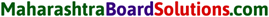Question c.
If …………….. of sound is decreased its loudness also decreases.
intensity

Question d.
A medium is necessary for …………… of sound.
generation

2. Match the pairs.

Question a.

 Column ‘A’ Column ‘B’ 1. Flute a. Frequency less than 20 Hz 2. Frequency b. Frequency more than 20,000 Hz 3. Sound level c. Vibrations in the air 4. Ultrasonic sound d. Measured in Hz 5. Infrasonic sound e. Decibel

 Column ‘A’ Column ‘B’ 1. Flute c. Vibrations in the air 2. Frequency d. Measured in Hz 3. Sound level e. Decibel 4. Ultrasonic sound b. Frequency more than 20,000 Hz 5. Infrasonic sound a. Frequency less than 20 Hz3. Give scientific reasons.

Question a.
In earlier times, people used to listen for the arrival of a distant train by putting their ear to the rails.

1. It was a technique that worked better in those days when train speed were slower than they are today and when there were more rail joints.
2. When people would put their ear to the rail they could hear vibration caused by the wheels moving over the joints. ,
3. Metal being a solid is an efficient medium for the sound to travel and so the sound can propagate further in the rail than would in air as speed of sound is faster in solids than in air.

Question b.
The sounds generated by a tabla and sitar are different.

1. In tabla where leather is fitted, sound gets generated when the leather surface is thumped by fingers or palm.
2. The waves thus created travel in two dimensions. Sitar is having strings attached to pumkin box called sound box.
3. Sounds generated by tabla and a sitar are different because they have different pitch and frequencies.

Question c.
If you were both on the moon your friend will not be able to hear you call.
Answer: Yes, your friend will not be able to hear your call because sound needs a medium for transmission since it is a mechanical wave it cannot travel through vaccum.

1. As there is no air on the moon and sound is actually vibration in air.
2. So astronauts communicate to each other by signals or microphones in space even when they are closer.Question d.
We can hear the movement of a mosquito wings but we cannot hear the movement of our hands.

1. Humans cannot hear frequency less than 20 Hz. In the movement of hands oscillations does take place but is less than frequency that humans can hear.
2. Mosquitos wings flap in air.
3. When they fly in air it produces buzzing sound in our ears.
4. The movement of mosquito wings produces sound greater than 20 Hz.
5. Sound is the reception of such waves and their perception by the brain.

4. Write answers to the following questions.

Question a.
How is sound produced?

1. Sound is generated due to the vibration of an object, e.g. bell, string or diaphragm of a musical instrument.
2. Vibrations are imparted to the molecules in the air. These vibrations reach our ears through the air and sound is heard.
3. Vibrations of an object is necessary for generation of sound.
4. These vibrations in air are called travelling longitudinal waves which we can hear.Question b.
What does the intensity of sound depend upon?

1. The intensity of sound is proportional to the square of the amplitude of vibration, e.g. If the amplitude is doubled the intensity of sound becomes four times.
2. Therefore the loudness or softness of sound is dependent on the intensity of sound and sound level.

Question c.
Explain how the frequency of oscillation is related to the length of a pendulum and the amplitude of its oscillation.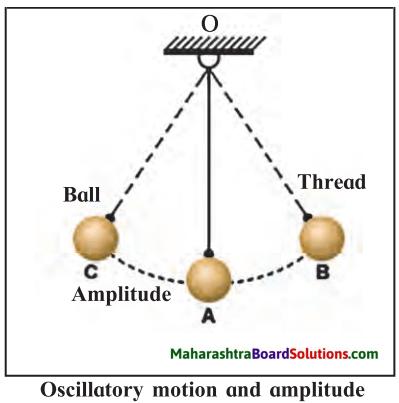1. Given an oscillatory motion to the pendulum, the maximum distance between the original position A of the pendulum and extreme position B or C is called the amplitude of oscillation. AB, AC is the amplitude of oscillation.
2. The number of oscillations completed by an oscillator in one second is called the frequency of oscillation.

Relation between frequency and length of a pendulum: If the length of the pendulum increases the period of oscillations also increases.
We know time period of oscillations = $$\frac{1}{\text { Frequency }}$$
If time period increases, frequency decreases, therefore, if the length of the pendulum increases frequency will decrease.
Relation between frequency and amplitude: Frequency of oscillation is independent of its amplitude of oscillation.Question d.
Explain the two ways by which the pitch of the sound generated by a stretched string can be changed?
1. A string vibrates with a particular fundamental frequency. It is however possible to produce pitches with different frequencies from the same string.

2. The two properties of the string that affect its frequency are length and diameter.

3. When the length of a string is changed, it will vibrate with a different frequency. Shorter strings have higher frequency and therefore higher pitch.

4. When a musician presses her finger on a string, she shortens its length. The more fingers she adds to the string, the shorter she makes it, higher will be the pitch.
Diameter: Thick strings with large diameter vibrate slower and have lower frequency than the thin ones.

Project:

Question a.
The bat, a mammal, flies during the night manoeuvering with the help of the ultrasonic sounds it produces. Find out more about this.

Class 7 Science Chapter 18 Sound: Production of Sound Important Questions and Answers

Fill in the blanks:

Question 1.
Bats detect the obstacles in their path by receiving the reflected ………….. .
ultrasonic wavesQuestion 2.
An object that moves back and forth is called ……………. .
an oscillator

Question 3.
If the length of a pendulum is increased the time period of oscillation ………………. .
increases

Question 4.
The …………… of a wave is the maximum distance moved by the object on either side of the mean position.
amplitude

Question 5.
Sound waves do not travel through ……………… .
vaccum

Question 6.
The frequency above which sound is not audible to the human ear is …………….. .
50,000 HzQuestion 7.
Number of vibrations in a second is called …………….. .
frequency

Question 8.
Higher the frequency greater will be the ………….. .
Pitch

Question 9.
The loudness of sound depends on ………….. .
amplitude

Question 10.
Loudness of sound is measured in units of ………………. .
decibel dBQuestion 11.
100 hertz is equal to hundred oscillations in ………………. .
one second

Name the following:

Question 1.
The sound frequencies above 20,000 Hz.
ultrasonic

Question 2.
The medium through which sound is most clearly propagated.
solidsQuestion 3.
The range of frequency in which humans can hear sound.
20 – 20,000 Hz

Question 4.
The sound level physically painful to humans.
above 100 dB

Question 5.
The technique used by bats to find their way.
echolocation

Question 6.
The unit of frequency of sound.
HertzQuestion 7.
The unit of sound level or intensity.
Decibel

Question 8.
The scientist in whose honour was the unit decibel named for intensity of sound.
Alexander graham Bell

Question 9.
Bats and dolphins can hear these sound frequencies.
Ultra sonic sound

Question 10.
The sound frequencies used in RADAR systems.
Ultra sonic sound

Answer in one or two sentences:

Question 1.
How is sound produced?
Sound is produced by vibration of an object.Question 2.
What produces sound in human beings ?
The vibration of the vocal chords in our larynx or voice box produces sound.

Question 3.
What is oscillatory motion?
To and fro motion of an object (oscillator) on either side of a central position is called oscillatory motion.

Question 4.
What is periodic motion?
The motion of an object repeated again and again at fixed intervals of time is called periodic motion.Question 5.
What is frequency of oscillation?
The number of oscillations completed by an oscillator in one second is called the frequency of oscillation.

Question 6.
How is intensity of sound and amplitude of vibration related?
The intensity of sound is proportional to the square of the amplitude of vibration. For example, if the amplitude is doubled the intensity becomes four times as much.

Question 7.
What happens if the frequency of sound is greater than 1000 Hz or 100 dB?
If frequency of sound is increased more than 100dB, the hearing can be temporarily affected which can result in temporary deafness.

Question 8.
What is infrasonic sound?
A sound with a frequency less than 20Hz is called infrasonic sound.Question 9.
What is application of ultrasonic sound?
Ultrasonic sound is used in ships as a system known as ‘SONAR’ to detect the obstacle beneath the ocean as well as the depth of an ocean.

Question 10.
What is the difference between pitch and frequency?
Pitch is directly proportional to the frequency of a sound whereas frequency is the repeating vibrations per unit time.

Question 11.
What happens when the rubber band is stretched with a greater force and released?
When a rubber is stretched with a greater force and released, the amplitude increases which in turn result in a louder sound being generated.Question 12.
What happens to frequency if the amplitude is changed?
The frequency remains the same even if the amplitude is increased or decreased.

Define:

1. Echo: It is a repetition of sound due to the reflection of original sound by a large and hard obstacle.
2. Frequency: It is defined as the number of vibrations per unit time.
3. Hertz (Hz): One hertz is one vibration per second.
4. Ultrasonic vibration: Sounds of frequency higher than 20,000 Hz are called the ultrasonic.
5. Time period: The time taken by an object to complete one oscillation is called time period.
6. Amplitude: The distance between the mean or central position to the extreme position of an oscillator on either side is called amplitude.

Say True or False with explanation:

Question 1.
Sound cannot travel in vacuum.
True. Sound needs a medium to travel through. Since vacuum does not provide any medium, sound cannot travel through it.

Question 2.
If the amplitude of vibration is large, sound is feeble.
False. When the amplitude of vibration of a sound is large, the sound is very loud. The sound is feeble, when the amplitude is small. Loudness of a sound is proportional to the square of the amplitude of its vibration.Question 3.
For human ears, the audible range is 2 Hz to 20,000 Hz.
True. Human being can hear sounds only within the range of 20-20,000 Hz. We cannot hear the sound having frequency out of this range.

Question 4.
The lower the frequency of vibrations the higher is the pitch.
False. Pitch of sound increases when the frequency of vibration increases. The pitch of a sound is directly proportional to its frequency.

Question 5.
Unwanted or unpleasant sound is termed as music.
False. Unwanted, or unpleasant sounds are termed noise. Sounds which are melodious, and pleasing to ears are called music.

Question 6.
Noise pollution may cause partial hearing impairment.
True. Unwanted, unpleasant sounds are called noise. If one is subjected to noise continuously for a long time, one may suffer from partial hearing impairment.Question 7.
Time taken by an object to complete one oscillation is called time period.
True. Time taken by an object to complete one oscillation is called time period.

Solve the following problems:

Question 1.
A pendulum oscillates 40 times in 4 seconds. Find its time period and frequency.
Frequency is the number of oscillations per second of a vibrating body.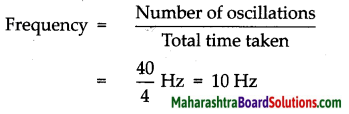The time taken to complete one oscillation (to and fro) is known, as time period.Question 2.
The sound from a mosquito, is produced when it vibrates its wings at an average rate of 500 vibrations per second. What is the time period of the vibration?
The time taken to complete one oscillation (to and fro) is know as time period.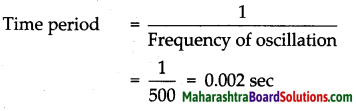Give scientific reasons:

Question 1.
Loud speakers should not be put in congested areas.

1. The loudness or softness is indicated by the intensity of sound and sound level.
2. By putting loud speakers in congested areas, the intensity of sound being more than 100 dB will cause ill effects like temporary deafness and a prolonged exposure can lead to permanent damage.Question 1.
Why are the ceilings of concert halls curved?
The ceilings of concert halls are curved because sound after reflection from the ceiling it reaches all the comers of the hall and is audible to each person in the hall.

Question 2.
Have you ever heard the sound of movement of hands and falling of leaves from a tree?

1. The term infrasonic refer to sound waves below the frequencies of audible sound i.e. under 20 Hz.
2. Humans cannot hear infrasonic sound.
3. Movement of hands and falling of leaves comes below this audible range. Therefore we cannot hear these sounds.

Question 3.
How can dogs perceive in advance the signals of an impending earthquake?
Dogs can hear sounds upto about 65,000 Hz. That’s why dogs can receive ultrasonic sound signals produced by the vibration of the earth and start barking in advance before the actual earthquake is felt.

Question 4.
State the uses of Ultrasonic sound.
Uses of Ultrasonic sound are:

1. To clean delicate ornaments and the tiny parts of a watch.
2. To observe internal organs of the body.
3. To detect tumors in the brain.
4. To detect faults in a metal.
6. To Kill certain microbes and insects.
7. SONAR (Sound navigation and ranging) is used to locate the seabed or the position of a ship.Question 1.
Will a sound be generated no matter how the ruler is kept on the table.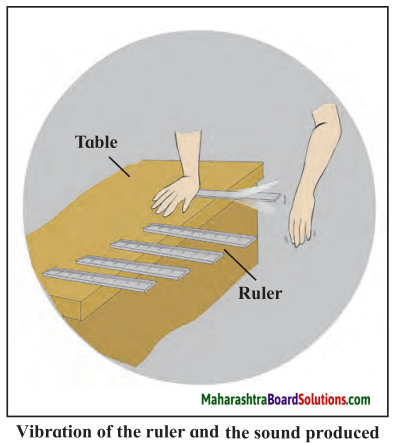1. No, If the ruler kept on the table is only a little part off the table, it will not vibrate with a greater force.
2. On the contrary if the ruler is kept with a larger portion off the table it will vibrate with a greater force generating louder sound.

Question 2.
Is there any correlation between the length of the free part of the ruler and the sound generated?
Yes, vibrating ruler pushes the molecules in the air producing sound waves. More the length of free part of ruler less will be the vibrations and less will be the frequency and pitch of the sound. If the length of free part of ruler is shorter it vibrates more quickly producing sound of higher frequency and pitch.Question 3.
If the ruler is plucked while it is held with 25 cm of it off the table, does it makes any sound? If there is no sound, look for the reason why it is so?